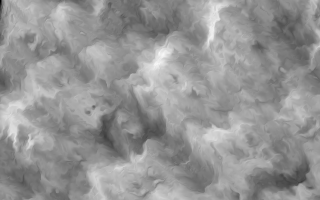Fractional Brownian Motion

# Fractional Brownian Motion

## Overview

Fractional Brownian Motion is a 2d noise algorithm used in computer graphics to produce textures.

Monolith implements an fBM based on shader code found in the Book of Shaders. When used with Domain Warping, fBM can be used to produce very complex textures out of a simple set of rules. When time is a factor, the textures can even move and swirl like some kind of cloudy liquid.

## Sample Code

The following Janet code uses `monolith/fbm` to render a 320x200 PNG image of a black and white fractal brownian motion texture.<<test_fbm.janet>>=
``````(def width 320)
(def height 200)
(def noctaves 5)
(def white @(255 255 255))
(def black @(0 0 0))

(monolith/gfx-fb-init)
(monolith/gfx-setsize 320 200)

(defn color [x y c]
(monolith/gfx-pixel-set
x y (math/floor (c 0)) (math/floor (c 1)) (math/floor (c 2)) 255))

(defn colorlerp [c1 c2 a]
(array
(+ (* a (c2 0)) (* (- 1 a) (c1 0)))
(+ (* a (c2 1)) (* (- 1 a) (c1 1)))
(+ (* a (c2 2)) (* (- 1 a) (c1 2)))))

(for ypos 0 height
(for xpos 0 width
(var x (/ xpos width))
(var y (/ ypos height))
(set x (* x (/ width height)))

(set y (* y 4))
(set x (* x 4))

(var fa (monolith/fbm x y noctaves))
(var fb (monolith/fbm (+ x fa) (+ y fa) noctaves))

(var amp (monolith/fbm (+ x fb) (+ y fb) noctaves))
(color xpos ypos (colorlerp black white amp))))

(monolith/gfx-write-png "fbm.png")``````

## A deeper dive

`monolith_fbm`, the core C function behind the Janet function `monolith/fbm` can be found in fbm.c in the section 4. Top-Level FBM Function.

Janet bindings can be found in fbm.c in the section 3. Janet Loader.